The CATMOD Procedure
 Repeated Measures Analysis

If there are multiple dependent variables and the variables represent repeated measurements of the same observational unit, then the variation among the dependent variables can be attributed to one or more repeated measurement factors. The factors can be included in the model by specifying _RESPONSE_ on the right side of the MODEL statement and by using a REPEATED statement to identify the factors.

To perform a repeated measures analysis, you also need to specify a RESPONSE statement, since the standard response functions (generalized logits) cannot be used. Typically, the MEANS or MARGINALS response functions are specified in a repeated measures analysis, but other response functions can also be reasonable.

### One Population

Consider an experiment in which each subject is measured at three times, and the response functions are marginal probabilities for each of the dependent variables. If the dependent variables each havelevels, then PROC CATMOD computes1 response functions for each time. Differences among the response functions with respect to these times could be attributed to the repeated measurement factor Time. To incorporate the Time variation into the model, specify the following statements:

```proc catmod;
response marginals;
model t1*t2*t3=_response_;
repeated Time 3 / _response_=Time;
run;
```

These statements produce a Time effect that has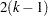degrees of freedom since there are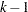response functions at each time point. For a dichotomous variable, the Time effect has two degrees of freedom.

Now suppose that at each time point, each subject has X-rays taken, and the X-rays are read by two different radiologists. This creates six dependent variables that represent the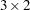cross-classification of the repeated measurement factors Time and Reader. A saturated model with respect to these factors can be obtained by specifying the following statements:

```proc catmod;
response marginals;
model r11*r12*r21*r22*r31*r32=_response_;
run;
```

If you want to fit a main-effects model with respect to Time and Reader, then change the REPEATED statement to the following:

```   repeated Time 3, Reader 2 / _response_=Time Reader;
```

If you want to fit a main-effects model for Time but for only one of the readers, the REPEATED statement might look like the following:

```   repeated Time \$ 3, Reader \$ 2
profile  =('1'  Smith,
'1'  Jones,
'2'  Smith,
'2'  Jones,
'3'  Smith,
'3'  Jones);
```

If Jones had been unavailable for a reading at time 3, then there would be onlyresponse functions, even though PROC CATMOD would be expecting some multiple of 6. In that case, the PROFILE= option would be necessary to indicate which repeated measurement profiles were actually represented:

```   repeated Time \$ 3, Reader \$ 2
profile  =('1'  Smith,
'1'  Jones,
'2'  Smith,
'2'  Jones,
'3'  Smith);
```

When two or more repeated measurement factors are specified, PROC CATMOD presumes that the response functions are ordered so that the levels of the rightmost factor change most rapidly. This means that the dependent variables should be specified in the same order. For this example, the order implied by the REPEATED statement is as follows, where the variable r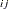corresponds to Timeand Reader:

Response

Dependent

Function

Variable

Time

1

r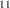1

1

2

r1

2

3

r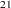2

1

4

r2

2

5

r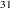3

1

6

r3

2

The order of dependent variables in the MODEL statement must agree with the order implied by the REPEATED statement.

### Multiple Populations

When there are variables specified in the POPULATION statement or on the right side of the MODEL statement, these variables produce multiple populations. PROC CATMOD can then model these independent variables, the repeated measurement factors, and the interactions between the two.

For example, suppose that there are five groups of subjects, that each subject in the study is measured at three different times, and that the dichotomous dependent variables are labeled t1, t2, and t3. The following statements computate three response functions for each population:

```proc catmod;
weight wt;
population Group;
response marginals;
model t1*t2*t3=_response_;
repeated Time / _response_=Time;
run;
```

PROC CATMOD then regards _RESPONSE_ as a variable with three levels corresponding to the three response functions in each population and forms an effect with two degrees of freedom. The MODEL and REPEATED statements tell PROC CATMOD to fit the main effect of Time.

In general, the MODEL statement tells PROC CATMOD how to integrate the independent variables and the repeated measurement factors into the model. For example, again suppose that there are five groups of subjects, that each subject is measured at three times, and that the dichotomous independent variables are labeled t1, t2, and t3. If you use the same WEIGHT, POPULATION, RESPONSE, and REPEATED statements as in the preceding program, the following MODEL statements result in the indicated analyses:

 model t1*t2*t3=Group / averaged; Specifies the Group main effect (with 4 degrees of freedom) model t1*t2*t3=_response_; Specifies the Time main effect (with 2 degrees of freedom) model t1*t2*t3=_response_*Group; Specifies the interaction between Time and Group (with 8 degrees of freedom) model t1*t2*t3=_response_|Group; Specifies both main effects, and the interaction between Time and Group (with a total of 14 degrees of freedom) model t1*t2*t3=_response_(Group); Specifies a Time main effect within each Group (with 10 degrees of freedom)

However, the following MODEL statement is invalid since effects cannot be nested within _RESPONSE_:

 model t1*t2*t3=Group(_response_);Previous Page | Next Page | Top of Page